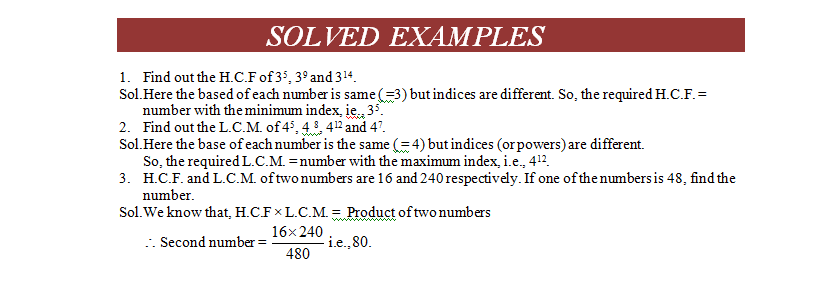# Difference between H.C.F. and L.C.M

## Lets find the Difference between H.C.F. and L.C.M

H.C.F. of x, y and z is the Highest Divisor which can exactly divide all of them

L.C.M of x. y and z is the Least Dividend which is exactly divisible by x, y and z.

## Co-primes:

Two numbers are said to be co-primes if their H.C.F. is 1.### H.C.F. and L.C.M. of Decimal Fractions:

In given numbers, make the same number of decimal places by annexing zeros in same numbers, if necessary. Considering these numbers without decimal point, find H.C.F or L.C.M. as the case may be. Now, in the result, mark off as many decimal places as are there in each of the given numbers.

### Solved Example of H.C.F. and L.C.M• The LCM. of two or more than two numbers is the greatest number that divides each of them exactly and that is always smaller or equal to those numbers.
• The least number which is exactly divisible by one of the given numbers is called their L.C.M.
• C.F. of numbers × L.C.M. of numbers = Product of numbers
• Two numbers are said to be co-primes if their L.C.M. is 1.
• The least number which is exactly divisible by 10,12,16 and 17 is 4080.